# Get Address of First Cell in Range

We will learn how to get address of first cell in range in this post. At Instance, we must determine the address of first cell in range to use as the data table’s value. For the DATA table, we have a range of value values in the form of an array that is sent to the formula as input, and therefore the formula returns the first cell value.

The next sections include information on the functions mentioned before that we will use.

ADDRESS retrieves the address of a cell using the row and column numbers.

## Syntax:

`=ADDRESS(row_num, col_num, [abs_num] )`
• `Row_num`: The Row number
• `Col_num`: The Column number
• `[abs num]`: [Optional] integer, one of the following values: 1, 2, 3, or 4. If this parameter is ignored or set to 1, the returned address will be absolute, e.g. \$A\$1.

Now we’ll combine these functions into a formula. We’ll begin with a data table. We need to determine the data table’s initial cell address.

Utilize the following formula:

`=ADDRESS(ROW(table_array), COLUMN(table_array))`

Variables:

• `table array`: array representation of a data table

## Examples:

Let us validate this formula by applying it to an example.

Here we have a data table and we need to use the formula to get the first cell address in the same data table.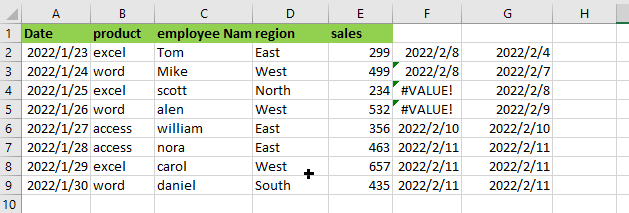Use the following Formula:

`= ADDRESS(ROW(A1:E9), COLUMN(A1:E9))`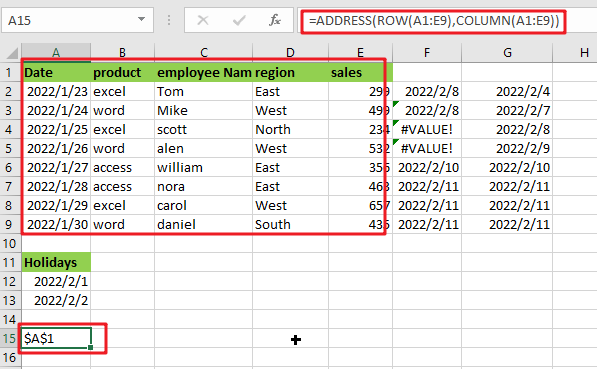Note: A1:E9 is array of tables

## Let’s See How This Formula Works:

`= COLUMN(A1:E9)`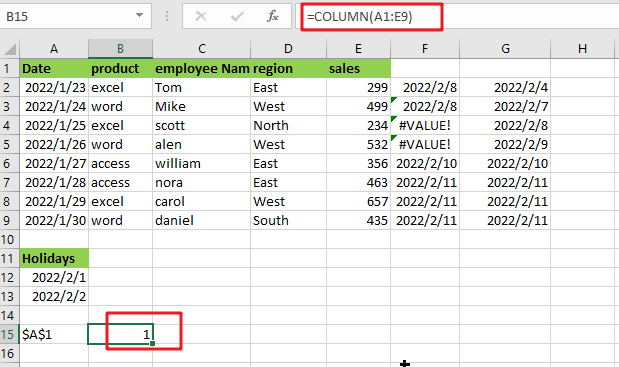The COLUMN function returns the value of the first column in the table array.

`= ROW(A1:E9)`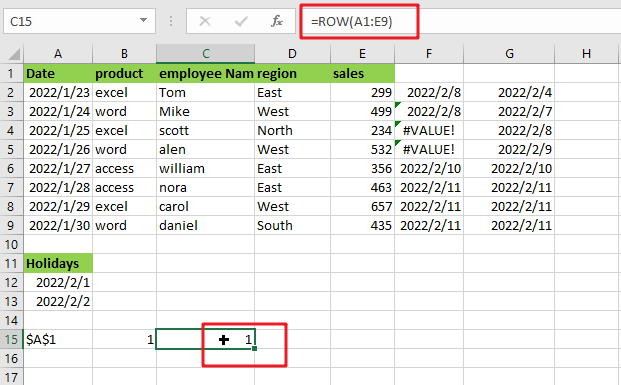The ROW function returns the index of the first row in the table array.

The ADDRESS function accepts the row number and column value parameters and returns the row’s and column’s absolute references.

The array sent to the function is denoted by cell reference. To get the result, press Enter.

As you can see, the formula works correctly since it delivers the value for the first cell in the data table.

Alternatively, you may use the CELL function:

CELL is an Excel built-in information function. Excel’s Cell function saves all the data in a specified cell and returns the cell’s info type. Using the follow formula:

`=CELL("address", [array_reference])`

or

`=CELL("address",INDEX(A1:E9,1,1))`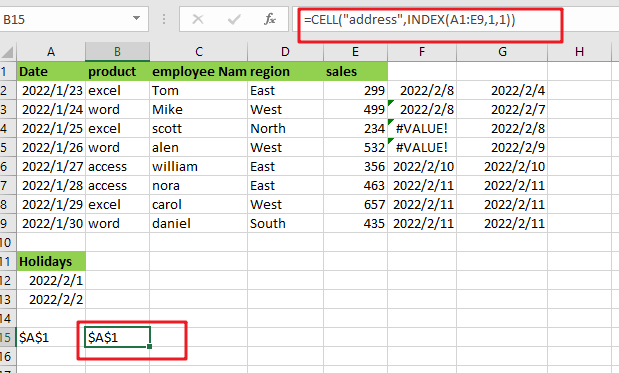Note:

• `[array_reference] `: table array given as reference.

As seen by the above calculation, you may use the first cell value as a reference.

The INDEX function can be used to get cell reference to the first cell in the given range by giving INDEX 1 for row number and 1 for column number.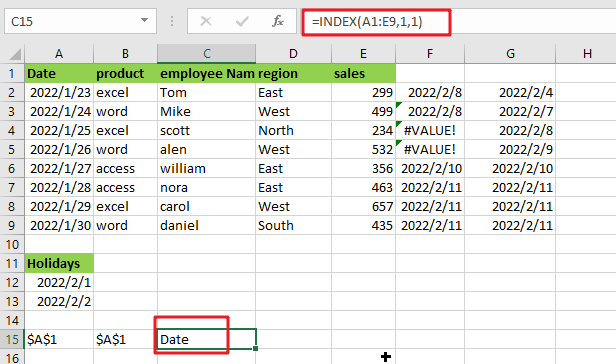The ADDRESS function may be used in conjunction with the ROW, COLUMN, and MIN functions to get the address of the first cell in a range. I hope this post on finding the get address of first cell in range in Excel was informative. It’s extremely easy and very helpful for people who use excel regularly.

### Related Functions

• Excel INDEX function
The Excel INDEX function returns a value from a table based on the index (row number and column number)The INDEX function is a build-in function in Microsoft Excel and it is categorized as a Lookup and Reference Function.The syntax of the INDEX function is as below:= INDEX (array, row_num,[column_num])…
• Excel ROW function
The Excel ROW function returns the row number of a cell reference.The ROW function is a build-in function in Microsoft Excel and it is categorized as a Lookup and Reference Function.The syntax of the ROW function is as below:= ROW ([reference])….